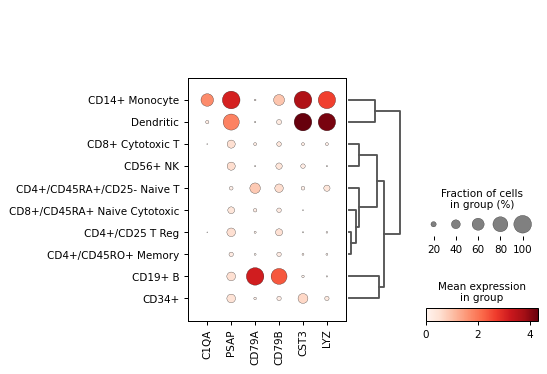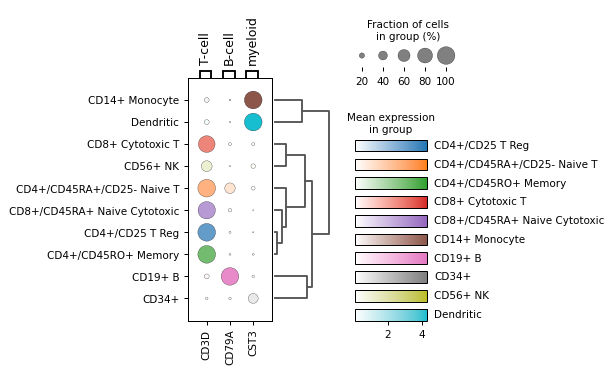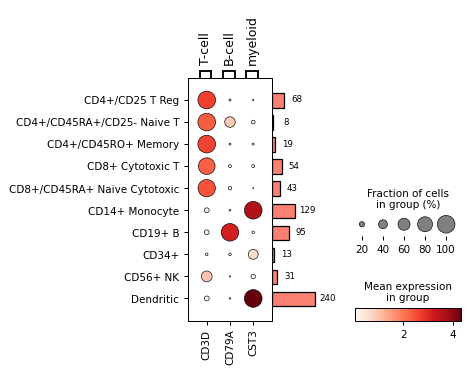# scanpy.pl.dotplot¶

scanpy.pl.dotplot(adata, var_names, groupby, use_raw=None, log=False, num_categories=7, expression_cutoff=0.0, mean_only_expressed=False, cmap='Reds', dot_max=None, dot_min=None, standard_scale=None, smallest_dot=0.0, title=None, colorbar_title='Mean expression\\nin group', size_title='Fraction of cells\\nin group (%)', figsize=None, dendrogram=False, gene_symbols=None, var_group_positions=None, var_group_labels=None, var_group_rotation=None, layer=None, swap_axes=False, dot_color_df=None, show=None, save=None, ax=None, return_fig=False, vmin=None, vmax=None, vcenter=None, norm=None, **kwds)

Makes a dot plot of the expression values of `var_names`.

For each var_name and each `groupby` category a dot is plotted. Each dot represents two values: mean expression within each category (visualized by color) and fraction of cells expressing the `var_name` in the category (visualized by the size of the dot). If `groupby` is not given, the dotplot assumes that all data belongs to a single category.

Note

A gene is considered expressed if the expression value in the `adata` (or `adata.raw`) is above the specified threshold which is zero by default.

An example of dotplot usage is to visualize, for multiple marker genes, the mean value and the percentage of cells expressing the gene across multiple clusters.

This function provides a convenient interface to the `DotPlot` class. If you need more flexibility, you should use `DotPlot` directly.

Parameters
adata : `AnnData``AnnData`

Annotated data matrix.

var_names :

`var_names` should be a valid subset of `adata.var_names`. If `var_names` is a mapping, then the key is used as label to group the values (see `var_group_labels`). The mapping values should be sequences of valid `adata.var_names`. In this case either coloring or ‘brackets’ are used for the grouping of var names depending on the plot. When `var_names` is a mapping, then the `var_group_labels` and `var_group_positions` are set.

groupby :

The key of the observation grouping to consider.

use_raw : (default: `None`)

Use `raw` attribute of `adata` if present.

log : `bool``bool` (default: `False`)

Plot on logarithmic axis.

num_categories : `int``int` (default: `7`)

Only used if groupby observation is not categorical. This value determines the number of groups into which the groupby observation should be subdivided.

categories_order

Order in which to show the categories. Note: add_dendrogram or add_totals can change the categories order.

figsize : (default: `None`)

Figure size when `multi_panel=True`. Otherwise the `rcParam['figure.figsize]` value is used. Format is (width, height)

dendrogram : (default: `False`)

If True or a valid dendrogram key, a dendrogram based on the hierarchical clustering between the `groupby` categories is added. The dendrogram information is computed using `scanpy.tl.dendrogram()`. If `tl.dendrogram` has not been called previously the function is called with default parameters.

gene_symbols : (default: `None`)

Column name in `.var` DataFrame that stores gene symbols. By default `var_names` refer to the index column of the `.var` DataFrame. Setting this option allows alternative names to be used.

var_group_positions : (default: `None`)

Use this parameter to highlight groups of `var_names`. This will draw a ‘bracket’ or a color block between the given start and end positions. If the parameter `var_group_labels` is set, the corresponding labels are added on top/left. E.g. `var_group_positions=[(4,10)]` will add a bracket between the fourth `var_name` and the tenth `var_name`. By giving more positions, more brackets/color blocks are drawn.

var_group_labels : (default: `None`)

Labels for each of the `var_group_positions` that want to be highlighted.

var_group_rotation : (default: `None`)

Label rotation degrees. By default, labels larger than 4 characters are rotated 90 degrees.

layer : (default: `None`)

Name of the AnnData object layer that wants to be plotted. By default adata.raw.X is plotted. If `use_raw=False` is set, then `adata.X` is plotted. If `layer` is set to a valid layer name, then the layer is plotted. `layer` takes precedence over `use_raw`.

title : (default: `None`)

Title for the figure

colorbar_title : (default: `'Mean expression\nin group'`)

Title for the color bar. New line character (n) can be used.

cmap : `str``str` (default: `'Reds'`)

String denoting matplotlib color map.

standard_scale : {‘var’, ‘group’} | `None``Optional`[`Literal`[‘var’, ‘group’]] (default: `None`)

Whether or not to standardize the given dimension between 0 and 1, meaning for each variable or group, subtract the minimum and divide each by its maximum.

swap_axes : (default: `False`)

By default, the x axis contains `var_names` (e.g. genes) and the y axis the `groupby` categories. By setting `swap_axes` then x are the `groupby` categories and y the `var_names`.

return_fig : (default: `False`)

Returns `DotPlot` object. Useful for fine-tuning the plot. Takes precedence over `show=False`.

size_title : (default: `'Fraction of cells\nin group (%)'`)

Title for the size legend. New line character (n) can be used.

expression_cutoff : `float``float` (default: `0.0`)

Expression cutoff that is used for binarizing the gene expression and determining the fraction of cells expressing given genes. A gene is expressed only if the expression value is greater than this threshold.

mean_only_expressed : `bool``bool` (default: `False`)

If True, gene expression is averaged only over the cells expressing the given genes.

dot_max : (default: `None`)

If none, the maximum dot size is set to the maximum fraction value found (e.g. 0.6). If given, the value should be a number between 0 and 1. All fractions larger than dot_max are clipped to this value.

dot_min : (default: `None`)

If none, the minimum dot size is set to 0. If given, the value should be a number between 0 and 1. All fractions smaller than dot_min are clipped to this value.

smallest_dot : (default: `0.0`)

If none, the smallest dot has size 0. All expression levels with `dot_min` are plotted with this size.

show : (default: `None`)

Show the plot, do not return axis.

save : (default: `None`)

If `True` or a `str`, save the figure. A string is appended to the default filename. Infer the filetype if ending on {`'.pdf'`, `'.png'`, `'.svg'`}.

ax : `_AxesSubplot` | `None``Optional`[`_AxesSubplot`] (default: `None`)

A matplotlib axes object. Only works if plotting a single component.

vmin : (default: `None`)

The value representing the lower limit of the color scale. Values smaller than vmin are plotted with the same color as vmin.

vmax : (default: `None`)

The value representing the upper limit of the color scale. Values larger than vmax are plotted with the same color as vmax.

vcenter : (default: `None`)

The value representing the center of the color scale. Useful for diverging colormaps.

norm : (default: `None`)

Custom color normalization object from matplotlib. See `https://matplotlib.org/stable/tutorials/colors/colormapnorms.html` for details.

kwds

Are passed to `matplotlib.pyplot.scatter()`.

Return type
Returns

If `return_fig` is `True`, returns a `DotPlot` object, else if `show` is false, return axes dict

`DotPlot`

The DotPlot class can be used to to control several visual parameters not available in this function.

`rank_genes_groups_dotplot()`

to plot marker genes identified using the `rank_genes_groups()` function.

Examples

Create a dot plot using the given markers and the PBMC example dataset grouped by the category ‘bulk_labels’.

```import scanpy as sc
markers = ['C1QA', 'PSAP', 'CD79A', 'CD79B', 'CST3', 'LYZ']
```Using var_names as dict:

```markers = {'T-cell': 'CD3D', 'B-cell': 'CD79A', 'myeloid': 'CST3'}
```Get DotPlot object for fine tuning

```dp = sc.pl.dotplot(adata, markers, 'bulk_labels', return_fig=True)```axes_dict = dp.get_axes()